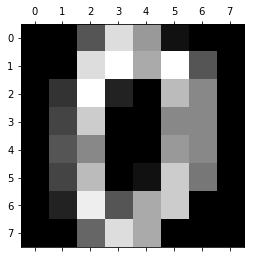# MNIST Digit Recognition

Before starting this tutorial, make sure you have `keras` and `sklearn` installed.

```\$ pip3 install keras sklearn
```

I've recently been getting into machine learning. One of the canonical problems in machine learning (particularly neural networks) is the MNIST dataset (Modified National Institute of Standards and Technology database); a collection of handwritten digits and their values.

Conveniently, this dataset is included in `sklearn`. However, the formatting is a little funky: we'll have to parse it more a bit later.

```from sklearn import datasets
```

Then, let's take a look at this dataset (always a good idea for any data):

```import matplotlib.pyplot as plt
plt.gray()
plt.matshow(digits.images)
```Let's split the data into an `X` and `Y` (in and out):

```X = digits.data
Y = digits.targets
```

Now, a few things to get this data ready for a neural network. First, we're going to want to format each of these images as a tensor, or a `n`-dimensional matrix (in this case `n=3`). For example:

```[
[ , , , ...],
[,  , , ...],
...
]
```

would be an image in our dataset. Each of these dimensions holds important information: the first separates rows in the image, the second separates pixels within each of these rows, and the third separates the components of the color of that pixel (usually red, green, and blue). Since the MNIST dataset is only black and white, we don't really use the last one; however it's something that Keras expects for image data.

Currently, the image data comes as a list of lists of pixels:

```[[0, 128, 30, ...], [12, 65, 19, ...], ...]
```

If we print `X.shape`, we'll get:

```(1797, 64)
```

The first dimension is the number of these lists, the second is the number of elements in each of these lists. The dimensions of our final array should have the same first dimension (`1797`), since it has the same amount of examples. However, we'll want to break this other dimension three other dimensions. To reshape it, we'll use this command:

```X = digits.data.reshape(-1, 1, 8, 8)
```

A `-1` in the `.reshape` method is a placeholder; it tells Numpy to not touch the `1797` dimension.

Neural networks use functions that operate in the range `[0, 1]`. Therefore, we'll have to fit the range of all numbers in `X` between `0` and `1`. Dividing by the maximum value will achieve this:

```X *= (1.0/np.max(X))
```

Now, time for some Python-fu. The outputs in the dataset are the actual numbers (e.g., `0, 1, 2, ...`). While one option for normalizing these data would be to divide each of these numbers by `9`, resulting in scalars between 0 and 1 (like above), neural networks will operate significantly better if you make the output as multidimensional vectors.

So, we'll be transforming scalars, like 3 or 9 to 10-dimensional normalized vectors like so:

```3 => [0, 0, 0, 1, 0, 0, 0, 0, 0, 0]
9 => [0, 0, 0, 0, 0, 0, 0, 0, 0, 1]
```

Here's the code I wrote:

```new_Y = []
for y in Y:
n =  * 10
n[y] = 1
new_Y.append(n)
Y = np.array(new_Y)
```

Finally, we'll have to convert this `Y` tensor from `int64` to `float64`:

```Y = Y.astype(np.float64)
```

Now, let's partition the data into a training set and a testing set. It's important to test the model on a separate dataset, since models can learn trends in datasets that aren't necessarily the actual thing we want to predict; therefore they may get good

```X_train = X[:-200]
Y_train = Y[:-200]

X_test = X[-200:]
Y_test = Y[-200:]
```

Now, let's configure the network in Keras. We'll be using a `Sequential` model.

```model = Sequential()
activation='relu',
input_shape=(1,8,8),
data_format='channels_first'))

rmsp = optimizers.RMSprop(lr=0.001)
model.compile(loss='logcosh', optimizer=rmsp, metrics=['accuracy'])
```

The last components here-the loss function and optimizer-can be tuned for better performance on particular datasets. It's good to try a few options for these.

Now, let's train the model:

```model.fit(X_train, Y_train, epochs=150, batch_size=10)
```

Finally, let's evaluate our model on the test portion of our dataset:

```print(model.evaluate(X_test, Y_test))
```

The first number it prints will be the loss, the second will be the accuracy.

Here's all the complete code:

```from sklearn import datasets
import matplotlib.pyplot as plt

plt.gray()
plt.matshow(digits.images)

X = digits.data
Y = digits.targets

X = digits.data.reshape(-1, 1, 8, 8)

X *= (1.0/np.max(X))

new_Y = []
for y in Y:
n =  * 10
n[y] = 1
new_Y.append(n)
Y = np.array(new_Y)
Y = Y.astype(np.float64)

X_train = X[:-200]
Y_train = Y[:-200]

X_test = X[-200:]
Y_test = Y[-200:]

model = Sequential()
activation='relu',
input_shape=(1,8,8),
data_format='channels_first'))